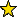# MultiplierIdeals -- multiplier ideals

## Description

MultiplierIdeals is a package for computing multiplier ideals, log canonical thresholds, and jumping numbers, using specialized routines wherever possible.

The package BernsteinSato provides computations of multiplier ideals, log canonical thresholds, and jumping numbers of arbitrary ideals using general algorithms.

This package provides alternatives for special classes of ideals, including monomial ideals, hyperplane arrangements, generic determinantal ideals, and binomial ideals (currently, ideals of curves in 3-space parametrized by monomials). These special computations are typically much faster than general methods and can often handle larger examples.

## References

• [BL] Blickle, Manuel and Robert Lazarsfeld, An informal introduction to multiplier ideals. Trends in commutative algebra, 87-114, Math. Sci. Res. Inst. Publ., 51, Cambridge Univ. Press, Cambridge, 2004.
• [H] Howald, J.A., Multiplier ideals of monomial ideals. Trans. Amer. Math. Soc. 353 (2001), no. 7, 2665-2671
• [J] Johnson, Amanda, Multiplier ideals of determinantal ideals. Thesis (Ph.D.)-University of Michigan. 2003
• [L] Lazarsfeld, Robert, Positivity in algebraic geometry. II. Ergebnisse der Mathematik., vol. 49, Springer-Verlag, Berlin, 2004. Positivity for vector bundles, and multiplier ideals.
• [M] Mustata, Mircea, Multiplier ideals of hyperplane arrangements. Trans. Amer. Math. Soc. 358 (2006), no. 11, 5015-5023.
• [T] Teitler, Zach, A note on Mustata's computation of multiplier ideals of hyperplane arrangements. Proc. Amer. Math. Soc. 136 (2008), no. 5, 1575-1579.
• [Th] Thompson, Howard M., Multiplier Ideals of Monomial Space Curves, Proc. Amer. Math. Soc. Ser. B 1 (2014), 33–41.

## Caveat

The multiplier ideals and log canonical thresholds computed by this package are valid over any field in any characteristic. In some cases results may be computed over other coefficient rings, such as ZZ, but they may not represent actual multiplier ideals or log canonical thresholds.

## CertificationVersion 1.1 of this package was accepted for publication in volume 7 of The Journal of Software for Algebra and Geometry on 5 June 2015, in the article Software for multiplier ideals. That version can be obtained from the journal or from the Macaulay2 source code repository.

## Version

This documentation describes version 1.1 of MultiplierIdeals.

## Source code

The source code from which this documentation is derived is in the file MultiplierIdeals.m2.

## For the programmer

The object MultiplierIdeals is .